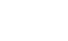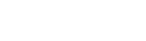# Kenya Certificate Of Secondary Education(KCSE 2013) Physics Paper 3 with Marking Scheme

Question 1. This question consists of two parts A and B: attempt both parts.
PART A.
You are provided with the following;

• A pendulum bob
• A stop-watch
• Two metre rules
• Two retort stands, two bosses and two clamps.

Proceed as follows:
1. Clamp one metre rule horizontally on the two stands so that the graduations are in a vertical plane. Suspend the pendulum bob from the metre rule with two pieces of thread so that the length of each thread from the point of support one the metre rule from the pendulum bob is 50 cm. See figure 1. The length of each thread will remain 50 cm throughout the experiment. The height of the metre rule above the bench should be at least 65 cm.2. Set the distance between A and B to be 70 cm. Displace the pendulum bob slightly in a plane perpendicular to the length of the metre rule and release it so that it oscillates in that plane. Measure and record in table 1 the time for 20 oscillations.
3. Repeat the procedure in (b) for other values of d shown in table 1. Complete the table.4.
1. Plot a graph T4 (y-axis) against d2. (4 marks)2. Determine the slopes of S on the graph. (2 marks)
3. Given that, determine the value of K. (2 marks)

PART B
You are provided with the following:
• Two stands, two clamps and two bosses
• One metre rule
• One Bar magnet
• Weighing balance (to be shared)
• Stop watch

Proceed as follows.
5. Using the metre rule as the length Land the breadth b for the magnet. (b is the second largest dimension on the magnet.)
L = ……………..m
b = ……………..m (1 mark)
6. Use the balance to measure the mass M of the magnet.
M = ……………kg. (1 mark)
7. Determine P given that P = M/3(L2 + b2). (2 marks)
8. Clamp the metre rule between the two retort stands. Using a piece of thread suspend the bar graph from the centre of the metre rule so that its length and breadth are both in a horizontal plane as shown in figure 2.
Keep away any unnecessary magnetic materials including voltmeter from this experimental set up.9.
1. Displace one end of the magnet through a small angle and let it oscillate about a vertical axis through its centre as shown by the arrows. Measure the time t for 10 oscillations.
t = ……………………s (1 mark)
2. Determine the period T for the oscillations. (1 mark)
3. Determine the constant G given that T= 2π√P/G (2marks)

Question 2
This question consists of two parts A and B, attempt both parts.

PART A
You are provided with a following

• a voltmeter
• a capacitor
• a switch
• a stop watch
• five connecting wires
• two cells and cell holder

Proceed as follows:

1. Connect the circuit as shown in figure 3.Make sure that the terminals of the capacitors and those of the battery are correctly connected.( positive to positive and negative to negative)
2. Close the switch, read and record the maximum voltage Vo across the capacitor.
Vo =…………………..volts (1 mark)
3. While the voltmeter shows the highest voltage Vo, open the switch and start the watch simultaneously. Stop the stopwatch when the voltage has dropped from Vo to 2.5 V. Read and record in table 2 the time taken.
4. Restart the stopwatch and close the switch. Repeat the procedure in (c) to measure and record the time taken for the voltage to drop from Vo to each of the other values shown in table 2.5.
1. On the grid provided, plot a graph of voltage V (y-axis) against time t.(4 marks)
2. Use the graph to determine the time t at which V= Vo/2
t = ………………seconds (1 mark)
6. Determine the resistance R of the voltmeter given that t = 0.693CR where C is the capacitance of the capacitor.

PART B
You are provided with the following:
• A triangular glass prism
• A metre rule
• A 50g mass
• Some hot water
• Some cold water
• One stand, one boss and one clamp
• A beaker

Proceed as follows:
7. Using a piece of thread suspend a metre rule from the clamp on the stand and adjust the position of the thread until the metre rule balances horizontally. Note this position O of the thread. (This position of the thread must be maintained throughout the experiment.)
8. Using another piece of thread suspend the glass prism from the metre rule at a point 35 cm from O. Suspend the 50 g mass on the opposite side of O using another piece of thread. Adjust the position of the thread attached to the 50 g mass until the metre rule balances once more. See figure 4.1. Determine the distance l1 between O and the point of support of the 50 g mass.
li= …………….cm (1 mark)
2. Use the principle of moments to determine the weight W1 of the prism in air. (1 mark)
9. Put cold water into the beaker (approximately three quarter 3/4 full). With the glass prism still at 35 cm from O, determine the distance l2 of the 50 g mass at which the rule balances when the prism is fully submerged in the cold water. See figure 51. l2 = ……………cm (1 mark)
2. Determine the weight W2 of the prism in the cold water. (1 mark)
10.
1. Measure and record the temperature T1 of the cold water when the system is balanced.
T1 = ………………..0C (1 mark)
2. Determine the distance l3 of the point of support of the 50 g mass when the prism is submerged in hot water.
l3 = …………….cm (1 mark)
11. Now pour out the cold water and replace it with hot water. Balance the metre rule with the prism fully submerged in hot water. Ensure that the prism is still supported at 35 cm from 0.
1. Measure and record the temperature T2 of the hot water.
T2 = ................... 0c (1 mark)
2. Determine the weight W3 of the prism in hot water.  (1 mark)
12. Determine the constant k for the water given that:
k =## MARKING SCHEME

PART A

1.
 Distance d (cm) 70 60 50 40 Time t for 20 oscillations(s) 24.3 25.8 26.7 27.5 Period T =  20  (s) 1.22 1.29 1.34 1.38 T(S ) 2.22 2.77 3.22 3.57 d (cm ) 4900 3600 2500 1600
Table 1 (6 marks)
2.
1. See graph (5 marks)
Scale and axis (1 marks)
Plotting (2 marks)
Line(1 mark)
2. Slope =  (422.5018)3.50  2  ;
3. K = √4.2 x 10-4;
=  963 S4cm-2; (3 marks)
3.1. PART B

1. l = 0.1 m
b = 0.01 m (1 mark)
2. m = 0.06 kg (1 mark)
3. p =  0.06 (0.12 + 0.012)
=  2.02 x 10-4 (2 marks)
1. t = 75s (1 mark)
2. T = 7.5s (1 mark)
3. 7.5 =  2r √2.02 x 10 - 4
G
G  = 1.42 x 10-4
unit not required

2. PART A

1. Vo = 3.0V (1 mark)
2.
 Voltage(V) 2.5 2.25 2 1.75 1.5 1.25 Time(s) 1.7 2.6 3.9 4.8 6.5 7.9
3.
1. see graph (5 marks)
2. t2  =6.4 S (1 mark)
4. R = 6.4 x 10 6
0.693 x 2200
= 4200 X (1 mark)

PART B

1.
1. L1 = 47.4 cm (1 mark)
2. W1 = 0.474 x 0.05 x 10
0.35
=  0.68 N (1 mark)
• W2 =
2. L= 28 cm (1 mark)
0.28 x 0.05 x 10
0.35
= 0.4 N ( 1 mark)
3. T1 = 26ºC
W3 =  0.285 x 0.05 x 10 (2 marks)
Accept (18 - 32ºC) (1 mark)
4.
1. L3 = 28.5 cm (1 mark)
2. T2 = 83ºC
Accept (60 - 95ºC) (1 mark)
0.35
=  0.41 (1 mark)
5. K = (0.68 - 0.4) - (0.68 - 0.41)
(0.68 - 0.41) (83 - 26)=  0.28 - 0.27
0.27 x 57
= 6.5  x 10-4K-1 (2 marks)

#### Download Kenya Certificate Of Secondary Education(KCSE 2013) Physics Paper 3 with Marking Scheme.

• ✔ To read offline at any time.
• ✔ To Print at your convenience
• ✔ Share Easily with Friends / Students